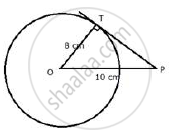Share

# The Radius of a Circle is 8 Cm. Calculate the Length of a Tangent Draw to this Circle from a Point at a Distance of 10 Cm from Its Centre. - Mathematics

Course

#### Question

The radius of a circle is 8 cm. calculate the length of a tangent draw to this circle from a point at a distance of 10 cm from its centre.

#### SolutionOP = 10 cm; radius OT = 8 cm

∵ OT ⊥ PT

in RT Δ OTP,

OP^2 = OT^2 +PT^2

10^2= 8^2 + PT^2

PT^2=100-64

PT^2=36

PT=6

Length of tangent = 6 cm.

Is there an error in this question or solution?